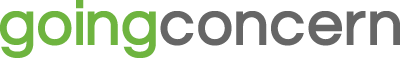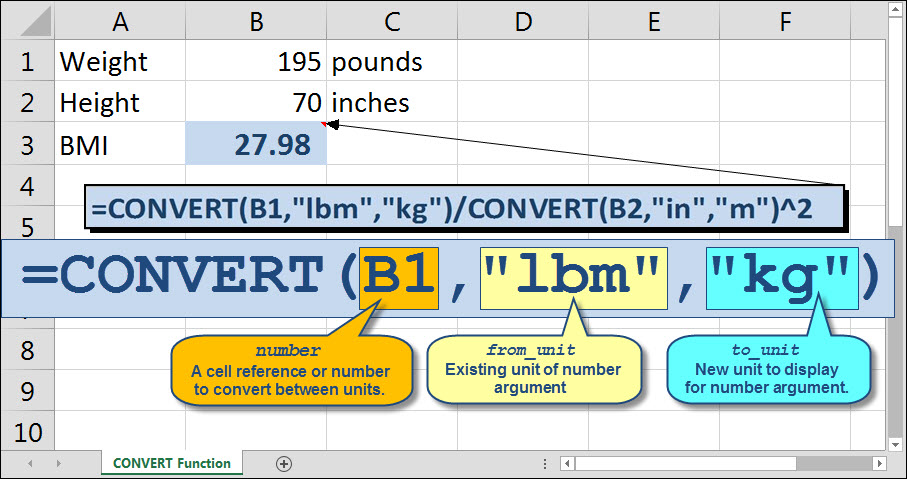April 10, 2020

#When accounting goes unaccounted for

Latest

# Use Excel to Keep an Eye on Your BMI This Busy Season

Let’s assume that you’ve bought into the quantified-self craze. Your wearable technology measures every breath you take and every move you make, so now it's time for new frontiers, as in something other than the fast food drive-thru lane. It’s always easiest to start with low-hanging fruit, such as your co-workers. Body Mass Index is a snap to calculate with Excel. You’re just two simple measurements and a worksheet function away. You’ll make Professor Don Tillman proud.

The first measurement you need is your height. If you've been slacking on your annual doctor's visit, either have a co-worker make you STAND UP STRAIGHT next to a wall or invite them to a Slurpee break and use the handy ruler on the convenience store’s doorframe. The second measurement you'll need is your weight. If you've been avoiding the scale for a while, no problem, you’re an accountant— make an estimate! The 10 pounds you put on every busy season is immaterial. For the purposes of this post, let’s use the average United States male height and weight of 195 pounds and 70 inches, respectively.

With these stats in hand you’re ready to fire up a blank Excel worksheet. As shown in Figure 1:

• Put the word Weight in cell A1, and then enter your pounds into cell B1.
• You can probably anticipate that height will go in the next row down. Use inches, just like the convenience store door does.

Now let’s pause for a bit of backstory. That treasure trove of medical knowledge known as the Internet shows a myriad of ways to calculate BMI. Most use rough approximations, but Excel will let us calculate BMIs out to fifteen decimal places. A commonly used BMI calculation involves converting one’s weight into kilograms, and height into meters. You then square the height and divide the result into the weight. It’s actually much easier than it sounds:

• Enter BMI into cell A3 and then this formula in cell B3:

=CONVERT(B1,"lbm","kg")/CONVERT(B2,"in","m")^2Figure 1: Use the CONVERT function to calculate BMI on the fly.

• Round off the decimals in cell B3 and you’ll see the average US male has a BMI of 27.98. Drop in the average US female’s weight/height of 165 pounds and 64 inches in cells B1 and B2 to return a BMI of 28.32.

Regardless, the CONVERT function is available in all versions of Excel and allows you to transform various measurements from one to another. You can probably surmise what abbreviations within the CONVERT function mean but I’ll spell them out just in case:

lbm – Pound Mass (as opposed to lbf for Pound Force)
kg – Kilograms (oddly this abbreviation won’t appear in the dropdown lists as you type the formula but it is a valid abbreviation)
in – Inches
m – Meters

The last two characters of the formula, “^2” square the result of the second CONVERT function. An Excel rookie would have taken this approach instead:

=CONVERT(B1,"lbm","kg")/(CONVERT(B2,"in","m")*(CONVERT(B2,"in","m"))

So there you have it, a new Excel function in your toolbox and some bits of meaningless information about the American public.

And here's a little bonus: you can put the formula to good use by converting the number of pints of beer you drink in a week to gallons. Enter your alcohol intake in cell A1 of a worksheet, and this formula in any other cell:

=CONVERT(A1,"us_pt","gal")

Whichever guilty pleasure — snacks or beer — you reach for a little too often this busy season, you can see how well (or not) you're handling it.